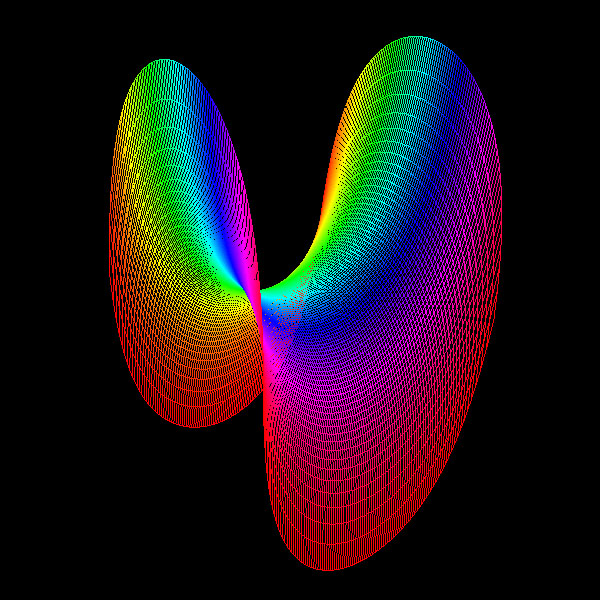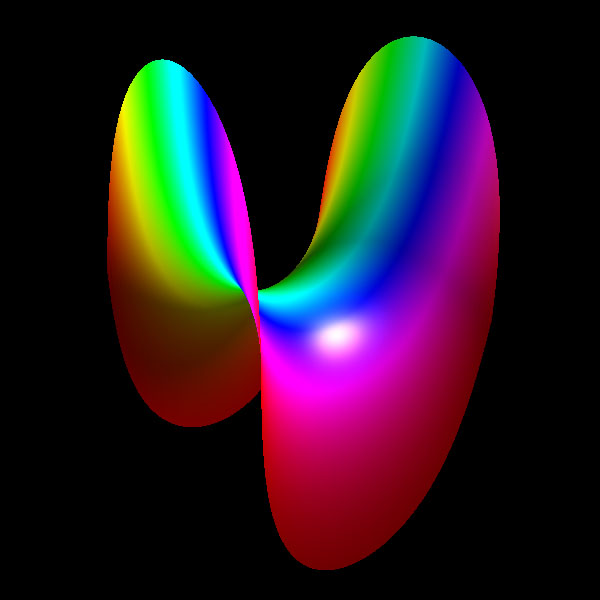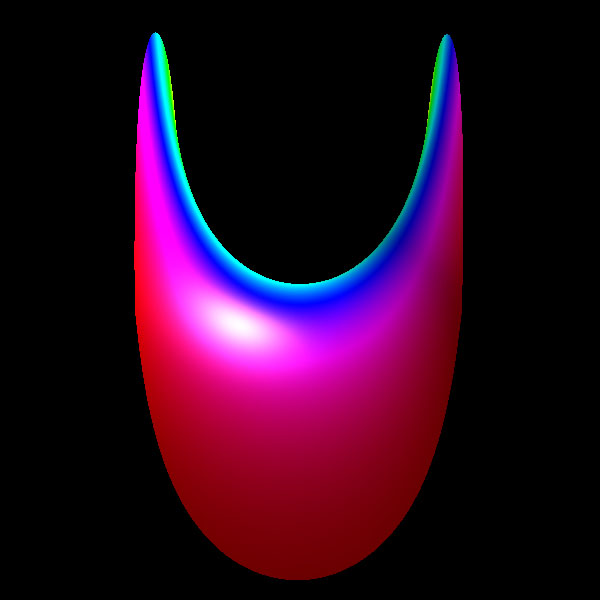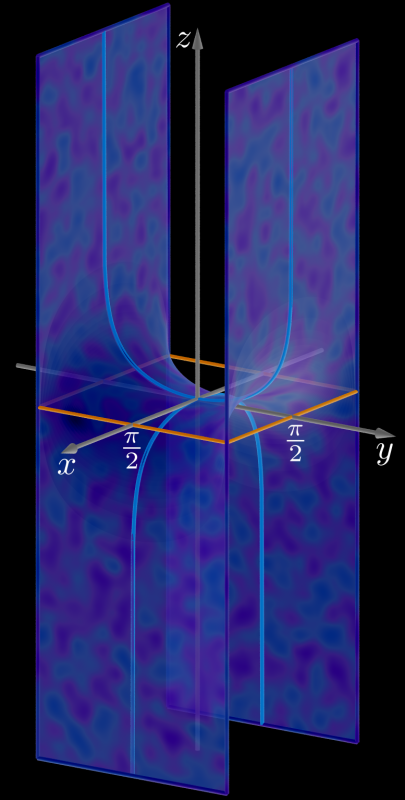# Scherk Minimal Surface

Written by Paul Bourke
December 2000
x = u
y = v
z = log(cos(c u) / cos(c v)) / c

Attributed to Heinrich Scherk circa 1835.
In the following three images the domain is

-pi <= u <= pi
-pi <= v <= piContribution by Jorge Delgado. POVRay source code: scherk.pov